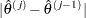RANDOM Statement

RANDOM variable </ options> ;
The RANDOM statement enables you to fit a shared frailty model for clustered data with normal distributed random effects (see the section The Frailty Model for details). The variable that represents the clusters must be a CLASS variable (declared in the CLASS statement). Currently, Bayesian analysis is not available for the frailty model.

The following options can be specified in the RANDOM statement:

ABSPCONV=r

specifies an absolute variance estimate convergence criterion for the doubly iterative estimation process. The PHREG procedure applies this criterion to the variance parameter estimate of the random effects. Supposedenotes the estimate of the variance parameter at theth optimization. By default, PROC PHREG examines the relative change in the variance estimate between optimizations (see the PCONV= option). The purpose of the ABSPCONV= criterion is to stop the doubly iterative process when the absolute changeis less than the tolerance criterion r. This convergence criterion does not affect the convergence criteria applied within any individual optimization. In order to change the convergence behavior within an individual optimization, you can use the ABSCONV=, ABSFCONV=, ABSGCONV=, ABSXCONV=, FCONV=, or GCONV= option in the NLOPTIONS statement.

ALPHA=value

specifies thelevel of the confidence limits for the random effects. The default is the value of the ALPHA= option in the PROC PHREG statement, or 0.05 if that option is not specified. This option is ignored if the SOLUTION option is not also specified.

METHOD=REML | ML

specifies the estimation method for the variance parameter. The REML specifications performs the residual maximum likelihood; this is the default method. The ML specification performs maximum likelihood.

NOCLPRINT

suppresses the display of the "Class Level Information for Random Effects" table.

PCONV=r

specifies the variance estimate convergence criterion for the doubly iterative estimation process. The PHREG procedure applies this criterion to the variance estimate of the random effects. Supposedenotes the estimate of variance at theth optimization. The procedure terminates the doubly iterative process if the relative changeis less than r. To check an absolute convergence criterion in addition, you can specify the ABSPCONV= option in the RANDOM statement. The default value for r is 1E–4. This convergence criterion does not affect the convergence criteria applied within any individual optimization. In order to change the convergence behavior within an individual optimization, you can use the ABSCONV=, ABSFCONV=, ABSGCONV=, ABSXCONV=, FCONV=, or GCONV= option in the NLOPTIONS statement.

SOLUTION

displays estimates of the normal random effects. Also displayed are estimates of the lognormal frailites, which are the exponentiated estimates of the normal random effects.

INITIALVARIANCE=value
INITIAL=value

specifies an inital value of the variance estimate. The default is INITIAL=1.